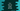# C++ program to invert all bits of a bitset variable## C++ program to invert all bits:

In C++, we can use compl to invert all bits of a bitset variable. This keyword makes it easy to invert the bits with just one line. In this post, I will show you how to use compl with one example.

### Syntax of compl:

Below is the syntax of compl:

``compl operand``

We can use this keyword with a bitset operand to invert all bits.

### C++ example program:

Below program shows how to use bitset:

``````#include <iostream>
#include <bitset>

using namespace std;

int main()
{
bitset<6> bitValue("101100");

cout << "Original value : " << bitValue << endl;

bitValue = compl bitValue;

cout << "After compl : " << bitValue << endl;
}``````

It prints the below output:

``````Original value : 101100
After compl : 010011``````